# Moran’s Eigenvector Maps and related methods for the spatial multiscale analysis of ecological data

#### 2021-04-06

The package adespatial contains functions for the multiscale analysis of spatial multivariate data. It implements some new functions and reimplements existing functions that were available in packages of the sedaR project hosted on R-Forge (spacemakeR, packfor, AEM, etc.). It can be seen as a bridge between packages dealing with multivariate data (e.g., ade4, Dray and Dufour (2007)) and packages that deals with spatial data (sp, spdep). In adespatial, many methods consider the spatial information as a spatial weighting matrix (SWM), object of class listw provided by the spdep package (Figure 1). The SWM is defined as the Hadamard product (element-wise product) of a connectivity matrix by a weighting matrix. The binary connectivity matrix (spatial neighborhood, object of class nb) defines the pairs of connected and unconnected samples, while the weighting matrix allows weighting the connections, for instance to define that the strength of the connection between two samples decreases with the geographic distance.

Once SWM is defined, it can be used to build Moran’s Eigenvector Maps (MEM, Dray, Legendre, and Peres-Neto (2006)) that are orthogonal vectors maximizing the spatial autocorrelation (measured by Moran’s coefficient). These spatial predictors can be used in multivariate statistical methods to provide spatially-explicit multiscale tools (Dray et al. 2012). This document provides a description of the main functionalities of the package.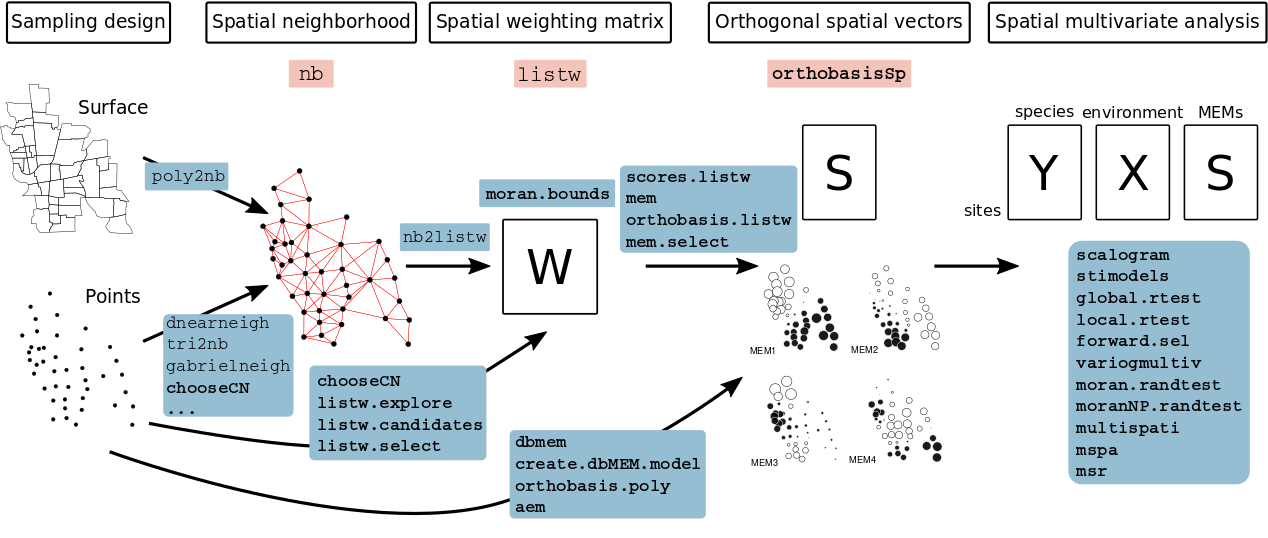Figure 1: Schematic representation of the functioning of the adespatial package. Classes are represented in pink frames and functions in blue frames. Classes and functions provided by adespatial are in bold.

To run the different analysis described, several packages are required and are loaded:

library(ade4)
library(adespatial)
## Registered S3 methods overwritten by 'adegraphics':
##   method         from
##   screeplot.dudi ade4
## Registered S3 method overwritten by 'spdep':
##   method   from
##   plot.mst ape
## Registered S3 methods overwritten by 'adespatial':
##   method             from
##   summary.multispati ade4
##
## Attaching package: 'adespatial'
## The following object is masked from 'package:ade4':
##
##     multispati
library(adegraphics)
##
## Attaching package: 'adegraphics'
## The following objects are masked from 'package:ade4':
##
##     kplotsepan.coa, s.arrow, s.class, s.corcircle, s.distri, s.image,
##     s.label, s.logo, s.match, s.traject, s.value, table.value,
##     triangle.class
library(spdep)
## Loading required package: sp
## Loading required package: spData
## To access larger datasets in this package, install the spDataLarge
## package with: install.packages('spDataLarge',
## repos='https://nowosad.github.io/drat/', type='source')
## Loading required package: sf
## Linking to GEOS 3.8.0, GDAL 3.0.4, PROJ 6.3.1
##
## Attaching package: 'spdep'
## The following object is masked from 'package:ade4':
##
##     mstree
library(maptools)
## Checking rgeos availability: TRUE

# 1 The Mafragh data set

The Mafragh data set is used to illustrate several methods. It is available in the ade4 package and stored as a list:

data("mafragh")
class(mafragh)
##  "list"
names(mafragh)
##   "xy"              "flo"             "neig"            "env"
##   "partition"       "area"            "tre"             "traits"
##   "nb"              "Spatial"         "spenames"        "Spatial.contour"
dim(mafragh$flo) ##  97 56 The data.frame mafragh$flo is a floristic table that contains the abundance of 56 plant species in 97 sites in Algeria. Names of species are listed in mafragh$spenames. The geographic coordinates of the sites are given in mafragh$xy.

str(mafragh$env) ## 'data.frame': 97 obs. of 11 variables: ##$ Clay        : num  0.73 0.75 0.74 0.23 0.73 0.72 0.52 0.42 0.74 0.53 ...
##  $Silt : num 0.24 0.24 0.24 0.26 0.24 0.22 0.46 0.49 0.24 0.44 ... ##$ Sand        : num  0.03 0.02 0.02 0.49 0.03 0.03 0.02 0.08 0.02 0.04 ...
##  $K2O : num 1.3 0.8 1.7 0.3 1.3 1.7 0.8 1.4 1.7 1.4 ... ##$ Mg++        : num  9.2 10.7 8.6 2 9.2 6 6 19.6 8.6 17 ...
##  $Na+/100g : num 4.2 10.4 10.8 1.2 4.2 10.7 18.4 2.5 10.8 11.7 ... ##$ K+          : num  1.2 1.4 1.9 0.3 1.2 1.3 2.2 1.3 1.9 0.5 ...
##  $Conductivity: num 7.9 11.5 10.4 0.6 7.9 14.5 15.2 2.9 10.4 16.9 ... ##$ Retention   : num  41.8 42.4 41.4 22.3 41.8 42.7 37.4 35.4 41.4 38 ...
##  $Na+/l : num 48.7 66 24 2.2 48.7 ... ##$ Elevation   : num  6 2 2 6 6 4 4 3 3 6 ...

The data.frame mafragh$env contains 11 quantitative environmental variables. A map of the study area is also available (mafragh$Spatial.contour) that can be used as a background to display the sampling design:

mxy <- as.matrix(mafragh$xy) rownames(mxy) <- NULL s.label(mxy, ppoint.pch = 15, ppoint.col = "darkseagreen4", Sp = mafragh$Spatial.contour)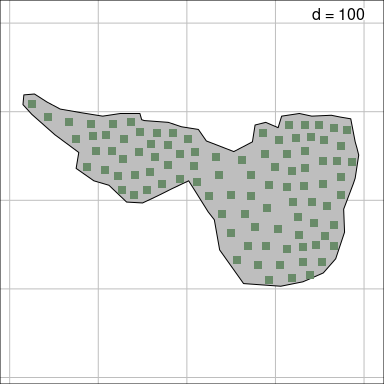A more detailed description of the data set is available at http://pbil.univ-lyon1.fr/R/pdf/pps053.pdf (in French).

The functionalities of the adegraphics package (Siberchicot et al. 2017) can be used to design simple thematic maps to represent data. For instance, it is possible to represent the spatial distribution of two species using the s.Spatial function applied on Voronoi polygons contained in mafragh$Spatial: mafragh$spenames[c(1, 11), ]
##                   scientific code
## Sp1         Arisarum vulgare Arvu
## Sp11 Bolboschoenus maritimus Boma
fpalette <- colorRampPalette(c("white", "darkseagreen2", "darkseagreen3", "palegreen4"))
sp.flo <- SpatialPolygonsDataFrame(Sr = mafragh$Spatial, data = mafragh$flo, match.ID = FALSE)
s.Spatial(sp.flo[,c(1, 11)], col = fpalette(3), nclass = 3)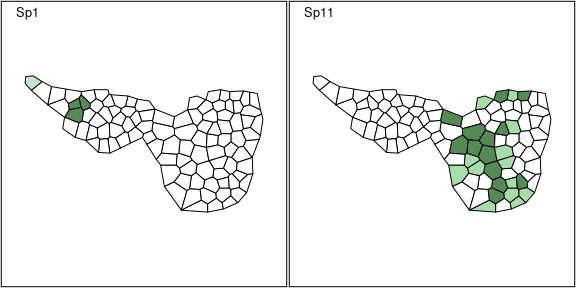# 2 Building spatial neighborhood

Spatial neighborhoods are managed in spdep as objects of class nb. It corresponds to the notion of connectivity matrices discussed in Dray, Legendre, and Peres-Neto (2006) and can be represented by an unweighted graph. Various functions allow to create nb objects from geographic coordinates of sites. We present different alternatives according to the design of the sampling scheme.

## 2.1 Surface data

The function poly2nb allows to define neighborhood when the sampling sites are polygons and not points (two regions are neighbors if they share a common boundary). The resulting object can be plotted on a geographical map using the s.Spatial function of the adegraphics package (Siberchicot et al. 2017).

data(mafragh)
class(mafragh$Spatial) ##  "SpatialPolygons" ## attr(,"package") ##  "sp" nb.maf <- poly2nb(mafragh$Spatial)
s.Spatial(mafragh$Spatial, nb = nb.maf, plabel.cex = 0, pnb.edge.col = 'red')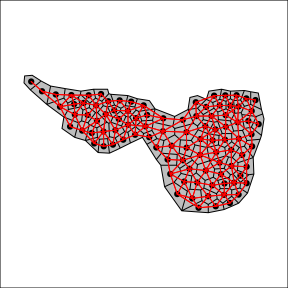## 2.2 Regular grid and transect If the sampling scheme is based on regular sampling (e.g., grid of 8 rows and 10 columns), spatial coordinates can be easily generated: xygrid <- expand.grid(x = 1:10, y = 1:8) s.label(xygrid, plabel.cex = 0)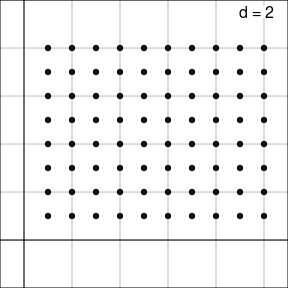For a regular grid, spatial neighborhood can be created with the function cell2nb. Two types of neighborhood can be defined. The queen specification considered horizontal, vertical and diagonal edges whereas the rook specification considered only horizontal and vertical edges: nb2.q <- cell2nb(8, 10, type = "queen") nb2.r <- cell2nb(8, 10, type = "rook") s.label(xygrid, nb = nb2.q, plabel.cex = 0, main = "Queen neighborhood")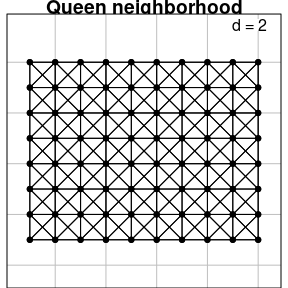s.label(xygrid, nb = nb2.r, plabel.cex = 0, main = "Rook neighborhood")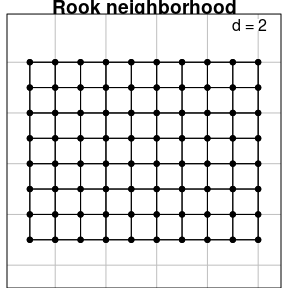The function cell2nb is the easiest way to deal with transects by considering a grid with only one row: xytransect <- expand.grid(1:20, 1) nb3 <- cell2nb(20, 1) summary(nb3) ## Neighbour list object: ## Number of regions: 20 ## Number of nonzero links: 38 ## Percentage nonzero weights: 9.5 ## Average number of links: 1.9 ## Link number distribution: ## ## 1 2 ## 2 18 ## 2 least connected regions: ## 1:1 1:20 with 1 link ## 18 most connected regions: ## 1:2 1:3 1:4 1:5 1:6 1:7 1:8 1:9 1:10 1:11 1:12 1:13 1:14 1:15 1:16 1:17 1:18 1:19 with 2 links All sites have two neighbors except the first and the last one. ## 2.3 Irregular sampling There are many ways to define the neighborhood in the case of irregular samplings. We consider a random subsample of 20 sites of the mafragh data set to better illustrate the differences between methods: set.seed(3) xyir <- mxy[sample(1:nrow(mafragh$xy), 20),]
s.label(xyir, main = "Irregular sampling with 20 sites")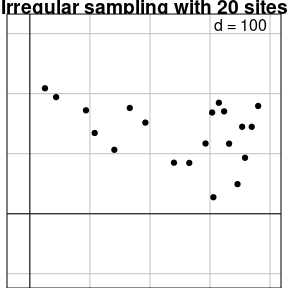The most intuitive way is to consider that sites are neighbors (or not) according to the distances between them. This definition is provided by the dnearneigh function:

nbnear1 <- dnearneigh(xyir, 0, 50)
nbnear2 <- dnearneigh(xyir, 0, 305)

g1 <- s.label(xyir, nb = nbnear1, pnb.edge.col = "red", main = "neighbors if 0<d<50", plot = FALSE)
g2 <- s.label(xyir, nb = nbnear2, pnb.edge.col = "red", main = "neighbors if 0<d<305", plot = FALSE)
cbindADEg(g1, g2, plot = TRUE)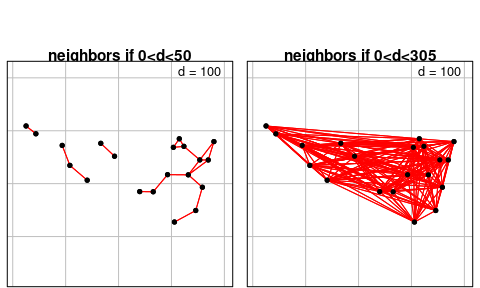Using a distance-based criteria could lead to unbalanced graphs. For instance, if the maximum distance is too low, some points have no neighbors:

nbnear1
## Neighbour list object:
## Number of regions: 20
## Number of nonzero links: 38
## Percentage nonzero weights: 9.5
## Average number of links: 1.9

On the other hand, if the maximum distance is too high, all sites are connected:

nbnear2
## Neighbour list object:
## Number of regions: 20
## Number of nonzero links: 354
## Percentage nonzero weights: 88.5
## Average number of links: 17.7

It is also possible to define neighborhood by a criteria based on nearest neighbors. However, this option can lead to non-symmetric neighborhood: if site A is the nearest neighbor of site B, it does not mean that site B is the nearest neighbor of site A.

The function knearneigh creates an object of class knn. It can be transformed into a nb object with the function knn2nb. This function has an argument sym which can be set to TRUE to force the output neighborhood to symmetry.

knn1 <- knearneigh(xyir, k = 1)
nbknn1 <- knn2nb(knn1, sym = TRUE)
knn2 <- knearneigh(xyir, k = 2)
nbknn2 <- knn2nb(knn2, sym = TRUE)

g1 <- s.label(xyir, nb = nbknn1, pnb.edge.col = "red", main = "Nearest neighbors (k=1)", plot = FALSE)
g2 <- s.label(xyir, nb = nbknn2, pnb.edge.col = "red", main = "Nearest neighbors (k=2)", plot = FALSE)
cbindADEg(g1, g2, plot = TRUE)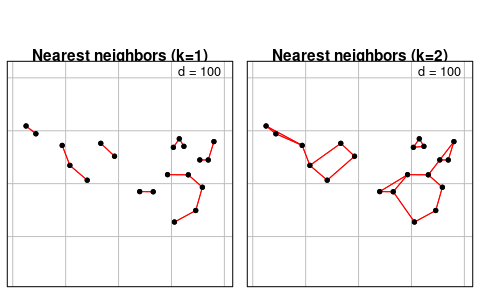This definition of neighborhood can lead to unconnected subgraphs. The function n.comp.nb finds the number of disjoint connected subgraphs:

n.comp.nb(nbknn1)
## $nc ##  7 ## ##$comp.id
##   1 2 3 1 4 5 3 6 2 3 3 7 1 6 5 4 7 5 7 3

More elaborate procedures are available to define neighborhood. For instance, Delaunay triangulation is obtained with the function tri2nb. It requires the package deldir. Other graph-based procedures are also available:

nbtri <- tri2nb(xyir)
nbgab <- graph2nb(gabrielneigh(xyir), sym = TRUE)
nbrel <- graph2nb(relativeneigh(xyir), sym = TRUE)

g1 <- s.label(xyir, nb = nbtri, pnb.edge.col = "red", main = "Delaunay", plot = FALSE)
g2 <- s.label(xyir, nb = nbgab, pnb.edge.col = "red", main = "Gabriel", plot = FALSE)
g3 <- s.label(xyir, nb = nbrel, pnb.edge.col = "red", main = "Relative", plot = FALSE)

ADEgS(list(g1, g2, g3))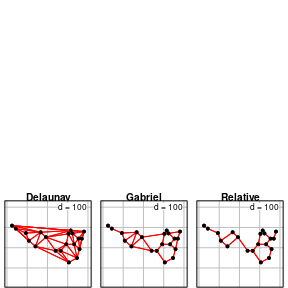The adespatial functions chooseCN and listw.candidates provides simple ways to build spatial neighborhoods. They are wrappers of many of the spdep functions presented above. The function listw.explore is an interactive graphical interface that allows to generate R code to build neighborhood objects (see Figure 2).

## 2.4 Manipulating nb objects

A nb object is not stored as a matrix. It is a list of neighbors. The neighbors of the first site are in the first element of the list:

nbgab[]
##   6 13

Various tools are provided by spdep to deal with these objects. For instance, it is possible to identify differences between two neighborhoods:

diffnb(nbgab, nbrel)
## Neighbour list object:
## Number of regions: 20
## Number of nonzero links: 14
## Percentage nonzero weights: 3.5
## Average number of links: 0.7
## 10 regions with no links:
## 4 5 7 9 10 11 16 17 18 20

Usually, it can be useful to remove some connections due to edge effects. In this case, the function edit.nb provides an interactive tool to add or delete connections.

The function include.self allows to include a site in its own list of neighbors (self-loops). The spdep package provides many other tools to manipulate nb objects:

intersect.nb(nb.obj1, nb.obj2)
union.nb(nb.obj1, nb.obj2)
setdiff.nb(nb.obj1, nb.obj2)
complement.nb(nb.obj)
nblag(neighbours, maxlag)

# 3 Defining spatial weighting matrices

A spatial weighting matrices (SWM) is computed by a transformation of a spatial neighborhood. We consider the Gabriel graph for the full data set:

nbgab <- graph2nb(gabrielneigh(mxy), sym = TRUE)

In R, SWM are not stored as matrices but as objects of the class listw. This format is more efficient than a matrix representation to manage large data sets. An object of class listw can be easily created from an object of class nb with the function nb2listw.

Different objects listw can be obtained from a nb object. The argument style allows to define a transformation of the matrix such as standardization by row sum, by total sum or binary coding, etc. General spatial weights can be introduced by the argument glist. This allows to introduce, for instance, a weighting relative to the distances between the points. For this task, the function nbdists is very useful as it computes Euclidean distance between neighbor sites defined by an nb object.

To obtain a simple row-standardization, the function is simply called by:

nb2listw(nbgab)
## Characteristics of weights list object:
## Neighbour list object:
## Number of regions: 97
## Number of nonzero links: 450
## Percentage nonzero weights: 4.782655
## Average number of links: 4.639175
##
## Weights style: W
## Weights constants summary:
##    n   nn S0      S1       S2
## W 97 9409 97 45.3915 395.3193

More sophisticated forms of spatial weighting matrices can be defined. For instance, it is possible to weight edges between neighbors as functions of geographic distances. In a fist step, distances between neighbors are obtained by the function :

distgab <- nbdists(nbgab, mxy)
nbgab[]
##  2 4 5 6
distgab[]
##  16.63971 21.34986 14.54966 16.99176

Then, spatial weights are defined as a function of distance (e.g. $$1-d_{ij}/max(d_{ij})$$):

fdist <- lapply(distgab, function(x) 1 - x/max(dist(mxy)))

And the spatial weighting matrix is then created:

listwgab <- nb2listw(nbgab, glist = fdist)
listwgab
## Characteristics of weights list object:
## Neighbour list object:
## Number of regions: 97
## Number of nonzero links: 450
## Percentage nonzero weights: 4.782655
## Average number of links: 4.639175
##
## Weights style: W
## Weights constants summary:
##    n   nn S0       S1     S2
## W 97 9409 97 45.41085 395.21
names(listwgab)
##  "style"      "neighbours" "weights"
listwgab$neighbours[] ##  2 4 5 6 listwgab$weights[]
##  0.2505174 0.2472375 0.2519728 0.2502723

The matrix representation of a listw object can also be obtained:

print(listw2mat(listwgab)[1:10, 1:10], digits = 3)
##     [,1]  [,2]  [,3]  [,4]  [,5]  [,6]  [,7]  [,8]  [,9] [,10]
## 1  0.000 0.251 0.000 0.247 0.252 0.250 0.000 0.000 0.000     0
## 2  0.250 0.000 0.250 0.000 0.000 0.250 0.250 0.000 0.000     0
## 3  0.000 0.251 0.000 0.000 0.000 0.000 0.251 0.249 0.249     0
## 4  0.199 0.000 0.000 0.000 0.201 0.200 0.000 0.000 0.000     0
## 5  0.503 0.000 0.000 0.497 0.000 0.000 0.000 0.000 0.000     0
## 6  0.201 0.201 0.000 0.199 0.000 0.000 0.202 0.000 0.000     0
## 7  0.000 0.200 0.200 0.000 0.000 0.201 0.000 0.199 0.000     0
## 8  0.000 0.000 0.167 0.000 0.000 0.000 0.167 0.000 0.167     0
## 9  0.000 0.000 0.333 0.000 0.000 0.000 0.000 0.335 0.000     0
## 10 0.000 0.000 0.000 0.000 0.000 0.000 0.000 0.000 0.000     0

To facilitate the building of spatial neighborhoods (nb object) and associated spatial weighting matrices (listw object), the package adespatial provides several tools. An interactive graphical interface is launched by the call listw.explore() assuming that spatial coordinates are still stored in an object of the R session (Figure 2).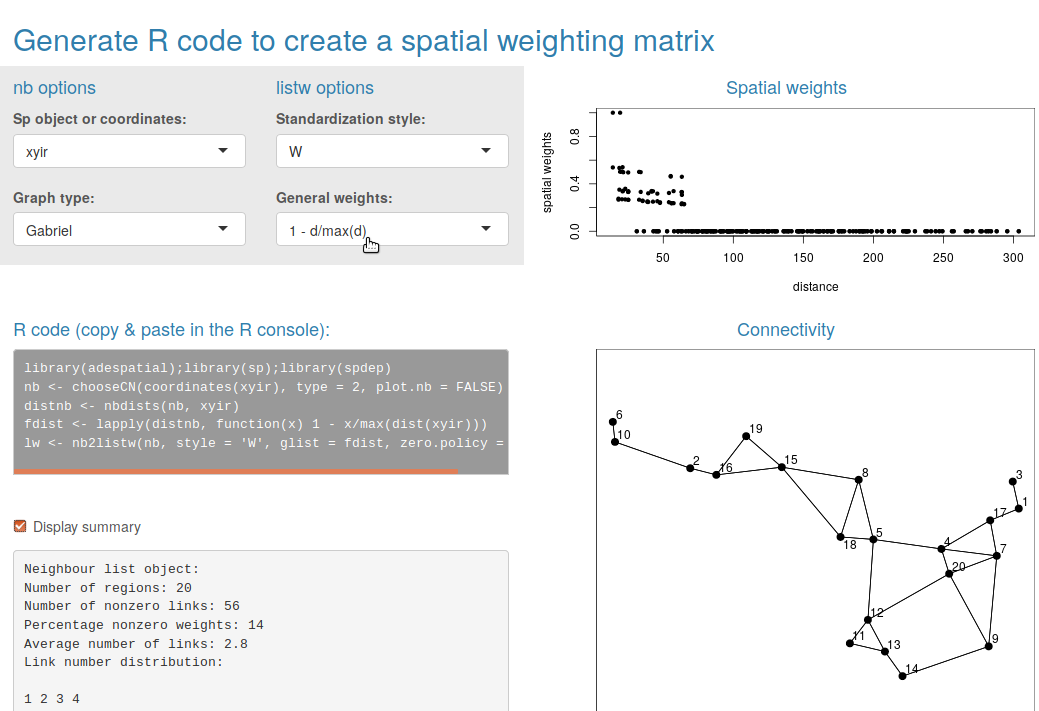Figure 2: The interactive interface provided by the function listw.explore.

# 4 Creating spatial predictors

The package adespatial provide different tools to build spatial predictors that can be incorporated in multivariate analysis. They are orthogonal vectors stored in a object of class orthobasisSp. Orthogonal polynomials of geographic coordinates can be computed by the function orthobasis.poly whereas principal coordinates of neighbour matrices (PCNM, Borcard and Legendre (2002)) are obtained by the function dbmem.

The Moran’s Eigenvectors Maps (MEMs) provide the most flexible framework. If we consider the $$n \times n$$ spatial weighting matrix $$\mathbf{W} = [w_{ij}]$$, they are the $$n-1$$ eigenvectors obtained by the diagonalization of the doubly-centred SWM:

$\mathbf{\Omega V}= \mathbf{V \Lambda}$

where $$\mathbf{\Omega} = \mathbf{HWH}$$ is the doubly-centred SWM and $$\mathbf{H} = \left ( \mathbf{I}-\mathbf{11}\hspace{-0.05cm}^{\top}\hspace{-0.05cm}/n \right )$$ is the centring operator.

MEMs are orthogonal vectors with a unit norm that maximize Moran’s coefficient of spatial autocorrelation (Griffith 1996; Dray et al. 2012) and are stored in matrix $$\mathbf{V}$$.

MEMs are provided by the functions scores.listw or mem of the adespatial package. These two functions are exactly identical (both are kept for historical reasons and compatibility) and return an object of class orthobasisSp.

mem.gab <- mem(listwgab)
mem.gab
## Orthobasis with 97 rows and 96 columns
## Only 6 rows and 4 columns are shown
##        MEM1      MEM2       MEM3        MEM4
## 1 0.9251530 -2.050270 -0.6159371  1.13648688
## 2 0.8495416 -1.859746 -0.4163876  0.57971608
## 3 0.8092292 -1.699300 -0.1970169 -0.02251458
## 4 1.0455937 -2.177654 -0.7488499  1.45727142
## 5 0.7098875 -1.571499 -0.5144638  1.00604362
## 6 0.9629486 -2.017900 -0.5572747  0.92335694

This object contains MEMs, stored as a data.frame and other attributes:

class(mem.gab)
##  "orthobasisSp" "orthobasis"   "data.frame"
names(attributes(mem.gab))
##  "names"     "class"     "row.names" "values"    "weights"   "call"

The eigenvalues associated to MEMs are stored in the attribute called values:

    barplot(attr(mem.gab, "values"),
main = "Eigenvalues of the spatial weighting matrix", cex.main = 0.7)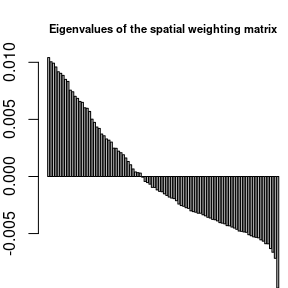A plot method is provided to represent MEMs. By default, eigenvectors are represented as a table (sites as rows, MEMs as columns). This representation is usually not informative and it is better to map MEMs in the geographical space by documenting the argument SpORcoords:

plot(mem.gab[,c(1, 5, 10, 20, 30, 40, 50, 60, 70)], SpORcoords = mxy)

or using the more flexible s.value function:

s.value(mxy, mem.gab[,c(1, 5, 10, 20, 30, 40, 50, 60, 70)], symbol = "circle", ppoint.cex = 0.6)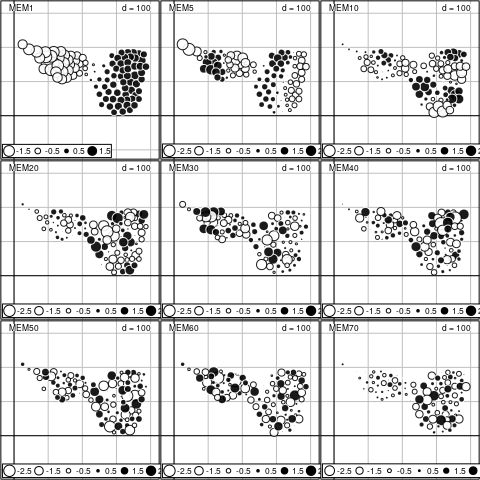Moran’s I can be computed and tested for each eigenvector with the moran.randtest function:

moranI <- moran.randtest(mem.gab, listwgab, 99)

As demonstrated in Dray, Legendre, and Peres-Neto (2006), eigenvalues and Moran’s I are equal (post-multiply by a constant):

head(attr(mem.gab, "values") / moranI$obs) ## MEM1.statistic MEM2.statistic MEM3.statistic MEM4.statistic MEM5.statistic ## 0.01030928 0.01030928 0.01030928 0.01030928 0.01030928 ## MEM6.statistic ## 0.01030928 # 5 Describing spatial patterns In the previous sections, we considered only the spatial information as a geographic map, a spatial weighting matrix (SWM) or a basis of spatial predictors (e.g., MEM). In the next sections, we will show how the spatial information can be integrated to analyze multivariate data and identify multiscale spatial patterns. The dataset mafragh available in ade4 will be used to illustrate the different methods. ## 5.1 Moran’s coefficient of spatial autocorrelation The SWM can be used to compute the level of spatial autocorrelation of a quantitative variable using the Moran’s coefficient. If we consider the $$n \times 1$$ vector $$\mathbf{x} = \left ( {x_1 \cdots x_n } \right )\hspace{-0.05cm}^{\top}\hspace{-0.05cm}$$ containing measurements of a quantitative variable for $$n$$ sites and $$\mathbf{W} = [w_{ij}]$$ the SWM. The usual formulation for Moran’s coefficient (MC) of spatial autocorrelation is: $MC(\mathbf{x}) = \frac{n\sum\nolimits_{\left( 2 \right)} {w_{ij} (x_i -\bar {x})(x_j -\bar {x})} }{\sum\nolimits_{\left( 2 \right)} {w_{ij} } \sum\nolimits_{i = 1}^n {(x_i -\bar {x})^2} }\mbox{ where }\sum\nolimits_{\left( 2 \right)} =\sum\limits_{i = 1}^n {\sum\limits_{j = 1}^n } \mbox{ with }i\ne j$ MC can be rewritten using matrix notation: $MC(\mathbf{x}) = \frac{n}{\mathbf{1}\hspace{-0.05cm}^{\top}\hspace{-0.05cm}\mathbf{W1}}\frac{\mathbf{z}\hspace{-0.05cm}^{\top}\hspace{-0.05cm}{\mathbf{Wz}}}{\mathbf{z}\hspace{-0.05cm}^{\top}\hspace{-0.05cm}\mathbf{z}}$ where $$\mathbf{z} = \left ( \mathbf{I}-\mathbf{1}\mathbf{1}\hspace{-0.05cm}^{\top}\hspace{-0.05cm}/n \right )\mathbf{x}$$ is the vector of centred values (i.e., $$z_i = x_i-\bar{x}$$). The function moran.mc of the spdep package allows to compute and test, by permutation, the significance of the Moran’s coefficient. A wrapper is provided by the moran.randtest function to test simultaneously and independently the spatial structure for several variables. sp.env <- SpatialPolygonsDataFrame(Sr = mafragh$Spatial, data = mafragh$env, match.ID = FALSE) maps.env <- s.Spatial(sp.env, col = fpalette(6), nclass = 6)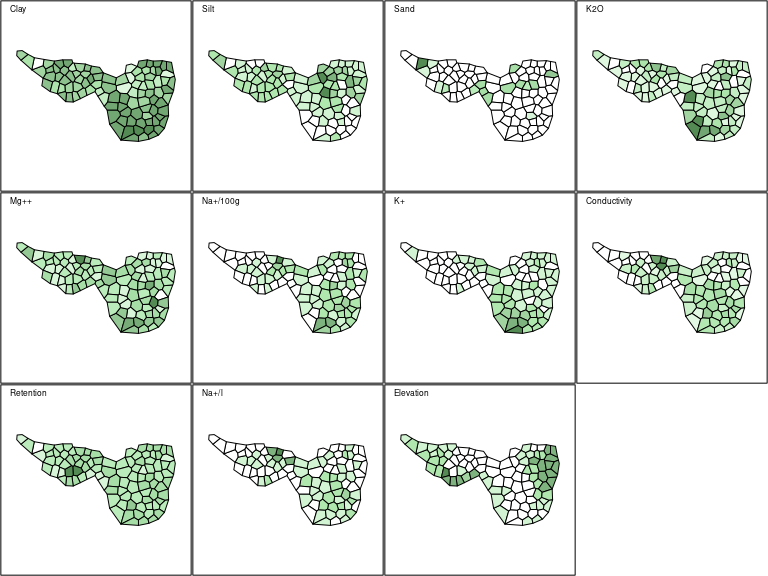MC.env <- moran.randtest(mafragh$env, listwgab, nrepet = 999)
MC.env
## class: krandtest lightkrandtest
## Monte-Carlo tests
## Call: moran.randtest(x = mafragh$env, listw = listwgab, nrepet = 999) ## ## Number of tests: 11 ## ## Adjustment method for multiple comparisons: none ## Permutation number: 999 ## Test Obs Std.Obs Alter Pvalue ## 1 Clay 0.4464655 6.552261 greater 0.001 ## 2 Silt 0.3967605 5.946167 greater 0.001 ## 3 Sand 0.1218959 2.114549 greater 0.033 ## 4 K2O 0.2916865 4.470113 greater 0.001 ## 5 Mg++ 0.2040580 3.220200 greater 0.003 ## 6 Na+/100g 0.3404142 5.138034 greater 0.001 ## 7 K+ 0.6696787 10.193028 greater 0.001 ## 8 Conductivity 0.3843430 5.891744 greater 0.001 ## 9 Retention 0.2217547 3.609803 greater 0.003 ## 10 Na+/l 0.3075238 4.791118 greater 0.001 ## 11 Elevation 0.6136770 9.047577 greater 0.001 For a given SWM, the upper and lower bounds of MC are equal to $$\lambda_{max} (n/\mathbf{1}\hspace{-0.05cm}^{\top}\hspace{-0.05cm}\mathbf{W1})$$ and $$\lambda_{min} (n/\mathbf{1}\hspace{-0.05cm}^{\top}\hspace{-0.05cm}\mathbf{W1})$$ where $$\lambda_{max}$$ and $$\lambda_{min}$$ are the extreme eigenvalues of $$\mathbf{\Omega}$$. These extreme values are returned by the moran.bounds function: mc.bounds <- moran.bounds(listwgab) mc.bounds ## Imin Imax ## -0.9474872 1.0098330 Hence, it is possible to display Moran’s coefficients computed on environmental variables and the minimum and maximum values for the given SWM: env.maps <- s1d.barchart(MC.env$obs, labels = MC.env$names, plot = FALSE, xlim = 1.1 * mc.bounds, paxes.draw = TRUE, pgrid.draw = FALSE) addline(env.maps, v = mc.bounds, plot = TRUE, pline.col = 'red', pline.lty = 3)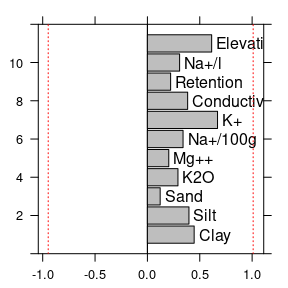## 5.2 Decomposing Moran’s coefficient The standard test based on MC is not able to detect the coexistence of positive and negative autocorrelation structures (i.e., it leads to a non-significant test). The moranNP.randtest function allows to decompose the standard MC statistic into two additive parts and thus to test for positive and negative autocorrelation separately (Dray 2011). For instance, we can test the spatial distribution of Magnesium. Only positive autocorrelation is detected: NP.Mg <- moranNP.randtest(mafragh$env[,5], listwgab, nrepet = 999, alter = "two-sided")
NP.Mg
## class: krandtest lightkrandtest
## Monte-Carlo tests
## Call: moranNP.randtest(x = mafragh$env[, 5], listw = listwgab, nrepet = 999, ## alter = "two-sided") ## ## Number of tests: 2 ## ## Adjustment method for multiple comparisons: none ## Permutation number: 999 ## Test Obs Std.Obs Alter Pvalue ## 1 I+ 0.3611756 3.743501 greater 0.002 ## 2 I- -0.1571176 1.552759 less 0.944 plot(NP.Mg)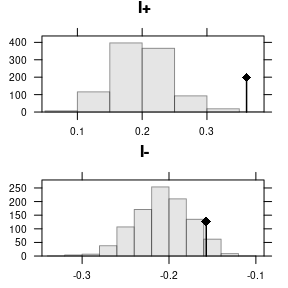sum(NP.Mg$obs)
##  0.204058
MC.env$obs ## Mg++.statistic ## 0.204058 ## 5.3 MULTISPATI analysis When multivariate data are considered, it is possible to search for spatial structures by computing univariate statistics (e.g., Moran’s Coefficient) on each variable separately. Another alternative is to summarize data by multivariate methods and then detect spatial structures using the output of the analysis. For instance, we applied a centred principal component analysis on the abundance data: pca.hell <- dudi.pca(mafragh$flo, scale = FALSE, scannf = FALSE, nf = 2)

MC can be computed for PCA scores and the associated spatial structures can be visualized on a map:

moran.randtest(pca.hell$li, listw = listwgab) ## class: krandtest lightkrandtest ## Monte-Carlo tests ## Call: moran.randtest(x = pca.hell$li, listw = listwgab)
##
## Number of tests:   2
##
## Adjustment method for multiple comparisons:   none
## Permutation number:   999
##    Test       Obs  Std.Obs   Alter Pvalue
## 1 Axis1 0.4830837 7.405295 greater  0.001
## 2 Axis2 0.4613738 7.119489 greater  0.001
s.value(mxy, pca.hell$li, Sp = mafragh$Spatial.contour, symbol = "circle", col = c("white", "palegreen4"), ppoint.cex = 0.6)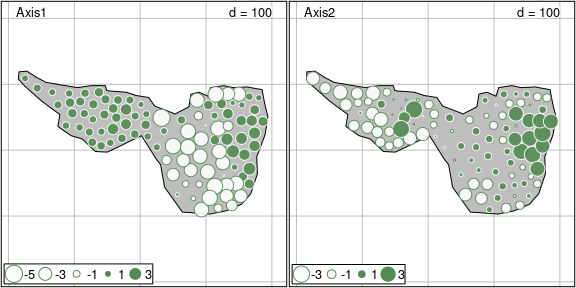PCA results are highly spatially structured. Results can be optimized, compared to this two-step procedure, by searching directly for multivariate spatial structures. The multispati function implements a method (Dray, Saïd, and Débias 2008) that search for axes maximizing the product of variance (multivariate aspect) by MC (spatial aspect):

ms.hell <- multispati(pca.hell, listw = listwgab, scannf = F)

The summary method can be applied on the resulting object to compare the results of initial analysis (axis 1 (RS1) and axis 2 (RS2)) and those of the multispati method (CS1 and CS2):

summary(ms.hell)
##
## Multivariate Spatial Analysis
## Call: multispati(dudi = pca.hell, listw = listwgab, scannf = F)
##
## Scores from the initial duality diagram:
##          var      cum     ratio     moran
## RS1 5.331174 5.331174 0.2834660 0.4830837
## RS2 1.972986 7.304159 0.3883725 0.4613738
##
## Multispati eigenvalues decomposition:
##          eig      var     moran
## CS1 2.933824 4.833900 0.6069269
## CS2 1.210573 1.892671 0.6396110

The MULTISPATI analysis allows to better identify spatial structures (higher MC values).

Scores of the analysis can be mapped and highlight some spatial patterns of community composition:

g.ms.maps <- s.value(mafragh$xy, ms.hell$li, Sp = mafragh$Spatial.contour, symbol = "circle", col = c("white", "palegreen4"), ppoint.cex = 0.6)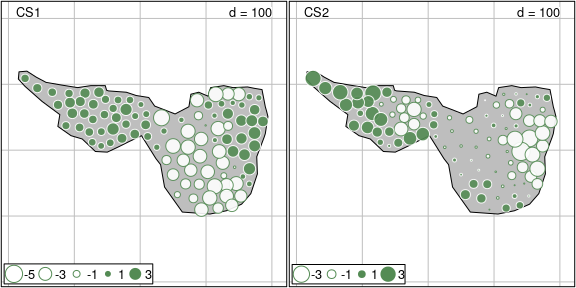The spatial distribution of several species can be inserted to facilitate the interpretation of the outputs of the analysis: g.ms.spe <- s.arrow(ms.hell$c1, plot = FALSE)
g.abund <- s.value(mxy, mafragh$flo[, c(12,11,31,16)], Sp = mafragh$Spatial.contour, symbol = "circle", col = c("black", "palegreen4"), plegend.drawKey = FALSE, ppoint.cex = 0.4, plot = FALSE)
p1 <- list(c(0.05, 0.65), c(0.01, 0.25), c(0.74, 0.58), c(0.55, 0.05))
for (i in 1:4)
g.ms.spe <- insert(g.abund[[i]], g.ms.spe, posi = p1[[i]], ratio = 0.25, plot = FALSE)
g.ms.spe